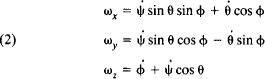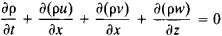# Euler's Equations

Also found in: Wikipedia.
The following article is from The Great Soviet Encyclopedia (1979). It might be outdated or ideologically biased.

## Euler’s Equations

(1) In mechanics, dynamical and kinematical equations used in the study of the motion of a rigid body. The equations were introduced by L. Euler in 1765.

Euler’s dynamical equations are differential equations of the motion of a body about a fixed point. They have the formwhere Ix, Iy, and Iz are the moments of inertia of the body about the principal axes of inertia (the origin of the frame of axes is the fixed point); ωx, ωy, and ωz are the components of the instantaneous angular velocity of the body about the principal axes; Mx, My, and Mz are the corresponding moments of the forces acting on the body; and ω̇x,ω̇y, and ω̇z are the components of the angular acceleration.

Euler’s kinematical equations give expressions for ωx, ωy, and ωz in terms of the Euler angles φ,ψ, and θ. They have the formThe system of equations (1) and (2) makes it possible to determine the moment of the forces acting on a body if the law governing the body’s motion is known. Conversely, the system permits determination of the law governing the motion if the forces acting on the body are known.

(2) In hydromechanics, the differential equations of motion of an ideal fluid in Eulerian variables. Let the pressure p, the density ρ, the components of the fluid-particle velocities u, v, and w, and the components of the body force X, Y, and Z be regarded as functions of the three space coordinates x, y, and z and of time t (Eulerian variables). Then Euler’s equations in rectangular Cartesian coordinates areThe solution of the general problem of hydromechanics in Eulerian variables reduces to determining u, v, w, p, and p as functions of x, y, z, and t if X, Y, and Z and the initial and boundary conditions are known. For this purpose, the equation of continuity in Eulerian variablesis appended to Euler’s equations.

In the case of a barotropic fluid, whose density depends solely on pressure, the fifth equation is the equation of state ρ = φ(p); p is constant if the fluid is incompressible.

Euler’s equations are used in solving various problems of hydromechanics.

### REFERENCES

Bukhgol’ts, N. N. Osnovnoi kurs teoreticheskoi mekhaniki, part 2, 9th ed. Moscow, 1972.
Subsecs. 14 and 16. Loitsianskii, L. G. Mekhanika zhidkosti i gaza, 4th ed. Moscow, 1973.

S. M. TARG